## Completion Of Incomplete Pattern

#### Completion Of Incomplete Pattern

Direction: Which answer figure will complete the question figure?

1. NA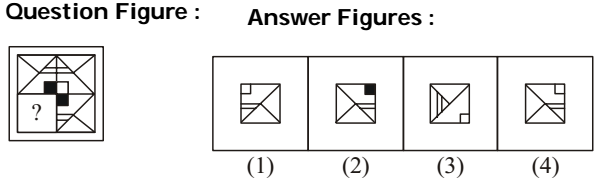1. NA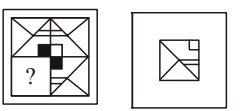##### Correct Option: D

NA1. NA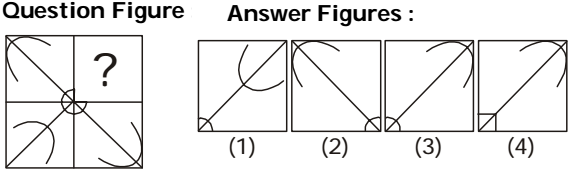1. NA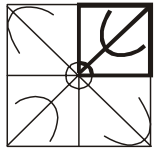##### Correct Option: A

NADirection: which answer figure will complete the pattern in the question figure?

1. NA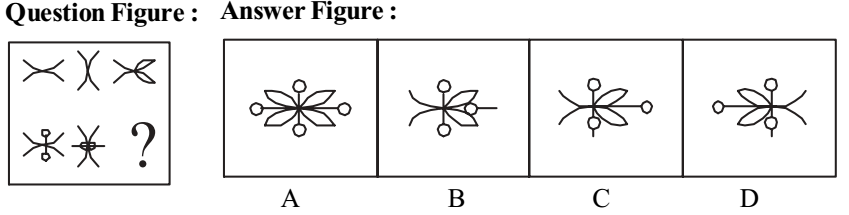1. NA

##### Correct Option: B

NA

Direction: In each of the following questions which one of the answer figures shall complete the given question figure.

1. NA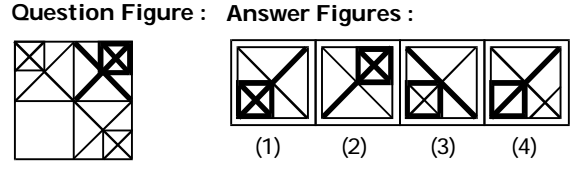1. The Answer figure (1) would complete the given figural pattern.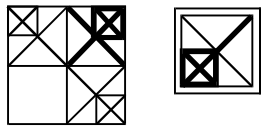##### Correct Option: A

The Answer figure (1) would complete the given figural pattern.1. NA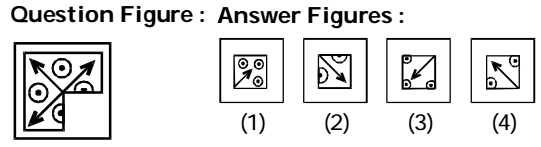1. NA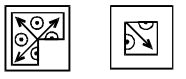##### Correct Option: B

NA